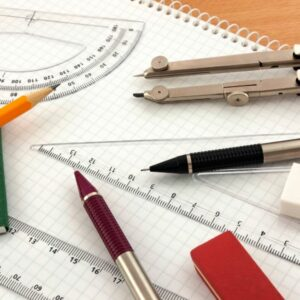##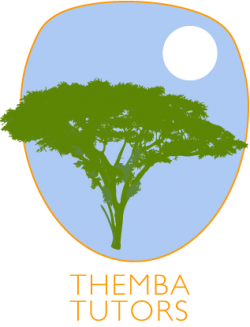Cornerstone Protection

836 Euclid Avenue Suite 16, Lexington

5.0 37 reviews

# NYC GEOMETRY TUTORING### Our GEOMETRY tutors travel to YOUR home:

Brooklyn Manhattan Queens Staten Island The Bronx

#### We also travel to:

Westchester New Jersey (NJ) Connecticut Long Island

## GEOMETRY TUTORING

### Module 1: Plane Geometry

#### Topic A: Introduction to Geometry

Plane Geometry is about flat shapes like lines, circles, and triangles … shapes that can be drawn on a piece of paper.
Point, Line, Plane and Solid
A Point has no dimensions, only position
A Line is one-dimensional
A Plane is two dimensional (2D)
A Solid is three-dimensional (3D)

Plane Geometry is all about shapes on a flat surface (like on an endless piece of paper).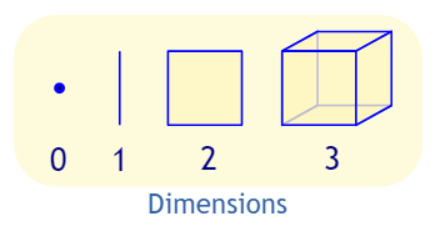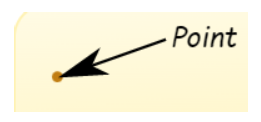A point really has no size at all! But we show them as dots so we can see where they are.

Now let’s allow the point to move in one direction. We get a line.We need just one value to find a point on that line. So we have one dimension. A line is one-dimensional.

Now let’s allow the point to move in a different direction.And we get a plane. We need two values to find a point on that plane. So we have two dimensions, or “2D”.
Circles, triangles, squares, and more are plane shapes.

We let that point move in another completely different direction, and we have three dimensions.Spheres, cubes, cylinders, and more are 3-dimensional or “3D”. We also call them solid shapes.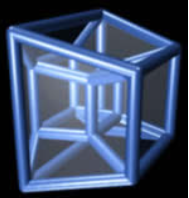The world we live in is 3D.
We can have more dimensions (such as this 4D Tessaract) in mathematics, but they are hard to draw!

#### 2D Shapes

A polygon is a plane (2D) shape with straight sides. To be a regular polygon, all the sides and angles must be the same:Triangle – 3 SidesHeptagon – 7 SidesSquare – 4 SidesOctagon – 8 SidesPentagon – 5 SidesNonagon – 9 SidesHexagon – 6 sidesDecagon – 10 Sides

#### Other Common Polygons

These are 2D shapes, and they are polygons, but not regular polygons:Any 4-sided 2D shapeRectangle – 4 Sides
All right angles

#### Curved Shapes

These are also 2D shapes but are not polygons because they have curves:CircleEllipse

#### Triangles

A triangle is a polygon with three edges and three vertices. It is one of the basic shapes in geometry. A triangle with vertices A, B, and C is denoted \triangle ABC. In Euclidean geometry, any three points, when non-collinear, determine a unique triangle and simultaneously, a unique plane. It can be classified into three: Equilateral, Isosceles, and Scalene.

There are three special names given to triangles that tell how many sides (or angles) are equal. There can be 3, 2, or no equal sides/angles:Equilateral Triangle
Three equal sides; Three equal angles, always 60°Isosceles Triangle
Two equal sides; Two equal angles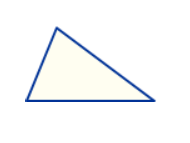Scalene Triangle
No equal sides; No equal angles

How to remember? Alphabetically they go 3, 2, none:

• Equilateral: “equal”-lateral (lateral means side) so they have all equal sides
• Isosceles: means “equal legs”, and we have two legs, right?   Also isosceles has two equal “Sides” joined by an “Odd” side.
• Scalene: means “uneven” or “odd”, so no equal sides.

#### What Type of Angle?

Triangles can also have names that tell you what type of angle is inside:Acute Triangle
All angles are less than 90°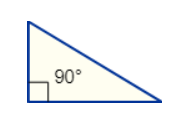Right Triangle
Has a right angle (90°)Obtuse Triangle
Has an angle more than 90°

#### Combining the Names

Sometimes a triangle will have two names, for example,Right Isosceles Triangle
Has a right angle (90°), and also two equal angles

### Topic B: Perimeter

Perimeter is the distance around a two-dimensional shape.

Example: the perimeter of this rectangle is 7+3+7+3 = 20Example: the perimeter of this regular pentagon is:3 + 3 + 3 + 3 + 3 = 5×3 = 15

The perimeter of a circle is called the circumference:#### Perimeter FormulasTriangle
Perimeter = a + b + cSquare
Perimeter = 4 × a
a = length of sideRectangle
Perimeter = 2 × (a + b)Perimeter = a + b + c + dCircle
Circumference = 2πr

Sector
Perimeter = r(θ+2)

Ellipse
Perimeter = very hard!

Topic C: Area

Area

Area is the quantity that expresses the extent of a region on the plane or a curved surface. The area of a plane region or plane area refers to the area of a shape or planar lamina, while surface area refers to the area of an open surface or the boundary of a three-dimensional object.Triangle
Area = ½ × b × h
b = base
h = vertical height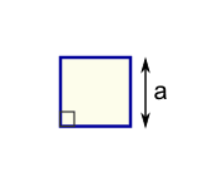Square
Area = a2
a = length of sideRectangle
Area = w × h
w = width
h = heightParallelogram
Area = b × h
b = base
h = vertical heightTrapezoid
Area = ½(a+b) × h
h = vertical heightCircle
Area = π × r2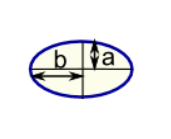Ellipse
Area = πabSector
Area = ½ × r2 × θNote: h is at right angles to b

Example: What is the area of this rectangle?The formula is:
Area = w × h
w = width
h = height

We know w = 5 and h = 3, so:
Area = 5 × 3 = 15
Example: What is the area of this circle?Area
= π × r2
= π × 32
= π × (3 × 3)
= 3.14159… × 9
= 28.27 (to 2 decimal places)

Example: What is the area of this triangle?Height = h = 12
Base = b = 20

Area = ½ × b × h = ½ × 20 × 12 = 120

Word Problem:
Example: Sam cuts grass at \$0.10 per square meter How much does Sam earn cutting this area:Let’s break the area into two parts:Part A is a square:
Area of A = a2 = 20m × 20m = 400m2
Part B is a triangle. Viewed sideways, it has a base of 20m and a height of 14m.
Area of B = ½b × h = ½ × 20m × 14m = 140m2
So the total area is:
Area = Area of A + Area of B = 400m2 + 140m2 = 540m2

Sam earns \$0.10 per square meter
Sam earns = \$0.10 × 540m2 = \$54

### MEET OUR GEOMETRY TUTORS WHO TRAVEL TO YOUR HOME#### BLAKE

High School Mathematics Teacher Completing Master’s Thesis and transitioning into his Doctoral Research at Teachers College, Columbia University#### MELISSA

Certified Math and Special Education Teacher, MS Math Education#### JOHN V.

Master’s in STEM Education and Master’s in Applied Math#### LEAH

Bachelor’s degree in Secondary Education with a Concentration in Mathematics, M.S. in General and Special Education (grades 1-6)#### DAVID

Master of Philosophy and Doctor of Philosophy, Master of Educational Leadership#### DANIEL

Master’s in Elementary Education, Bachelor’s in Political Science & French

#### EVAN

Master’s in Leadership in Math Education, Bachelor’s Degree in Mathematics#### BASSEM

M.A degree in Clinical Psychology

Chat with Themba Tutors Today!
Our Geometry Tutors work with all skill levels, travel to you, and offer remote tutoring services

FREE CONSULTATION WITH A GEOMETRY TUTOR!!!

Call: (917) 382-8641, Text: (833) 565-2370

Email: info@thembatutors.com

(we respond to email right away!).

##### References:

https://www.mathsisfun.com/geometry/index.html

The following two tabs change content below.

#### Craig Selinger

Owner at Themba Tutors
Craig Selinger, CEO of Themba Tutors (serving Manhattan, Brooklyn, Queens, Staten Island, Bronx, Westchester, Long Island, New Jersey, and Connecticut), is a NY State licensed speech-language pathologist, executive functioning coach, and learning specialist with over 18 years of experience working professionally with over a thousand families. His expertise includes language-based learning issues, e.g. reading, writing, speaking, and listening, executive functioning, ADHD/ADD, and learning disabilities. Check out his interviews with top-notch professionals in the field on Spotify.
Share: